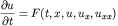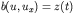# Solving Partial Differential Equations with Octave

## PDECOL

This is the first release of some code I have written for solving one-dimensional partial differential equations with Octave. The types of equations that can be solved with this method are of the following formHere u can be a vector of unknowns depending on both space and time. The spatial domain x is finite i.e. [a,b]. Here u_x is the first partial derivative of the vector of unknowns and u_xx is the vector of second partial derivatives, u_t is the vector of time derivatives. Boundary conditions depend on the partial differential equation (PDE) solved and are imposed in the octave code as equations of the following formHere b and z are user defined vector valued functions. The initial conditions are specified for each component of u at the initial time t_0. These initial conditions must be consistent with the boundary conditions above. The user must specify octave functions (with a very similar format to Matlab functions) defining each of the functions described above for the specific problem at hand. As such it is very easy to modify and solve various different PDES. One can use the same functional call and just change the octave functions references. Various examples of this use are provided in the software below. The code is currently a C++/octave API wrapper that calls the core solution routine PDECOL described in the paper:

Algorithm 540: PDECOL, General Collocation Software for Partial Differential Equations
by N. K. Madsen, R. F. Sincovec
ACM Transactions on Mathematical Software (TOMS), Volume 5 Issue 3 (September 1979)

The algorithm is based on the method of lines and uses a finite element collocation procedure with B-splines as its basis elements. The polynomial order of the splines is an input parameter. The allowable values are from (2 to 20). The ordinary differential equations that result from a B-spline basis and the imposed collocation can be integrated with either of two main methods: Adams' methods (of order between 1 and 12) and backwards differentiation methods (of order between 1 and 5).

## Examples:

• The first example from the Madsen and Sincovec paper (using octave) is given here. This is a rather complicated nonlinear system of partial differential equations. In addition, included in the source code below, are all of the additional examples from this paper.
• If anyone finds this software helpful please send me an example of its use, I would be happy to put it here.

## Misc:

The license in the ACM code only allows non-commercial usage of their codes. This license is too restrictive for the Free Software Foundation GNU copyleft and therefore the code cannot be included in the mainstream Octave sources. This license does however permit usage of this software for educational purposes.

Please reference this software in any publications that result from its use. A sample bibtex entry is below

``` @misc{weatherwaxPDECOLG, author = "J L. Weatherwax", title = "Software for solving PDE's with Octave", text = "PDECOLG: An Octave Gateway Routine to pdecol.f", year = "2005", url = "http://web.mit.edu/wax/www/Software/pdecol.html" } ```

I should mention that I am very interested in having people use this software and as such would be very willing to help get people started using it. As always, please send any comments to the address below.
• The version 1.2 has all working examples from the Madsen and Sincovec paper.
• The version 1.1 has a few minor bugs fixed over version 1.0. Specifically, the C++ code was modified to return the initial condition along with the solution at the requested time. Previous version didn't return the initial condition.
• The version 1.0 code is effectively finished. It has had several enhancements to improve readability and functionality of the sections of the code users will interact with and more extensive verification using of many of the examples from the paper above. These examples are included in this release.
• The version 0.9 code now allows the specified PDE to be define as octave functions. In addition, the directory EG1 has a simple example of its use.
• The version 0.1 code does work but is rather primitive in many ways. The main restriction is that it still requires the user code his/her partial differential equations, initial conditions, and boundary conditions as described above in FORTRAN. This version does allow easy parameter variations such as the number of grid points, and does all memory allocation once the problem definition FORTRAN is coded. The requirement of FORTRAN is a major drawback. I've decided to release this anyways and begin development on a version that will not require any FORTRAN coding. This should be coming shortly.

## Installation

### General Notes:

The code was compiled on a 686 athalon chip and thus should not need to be recompiled if you are using a similar chipset. If this is not the case to rebuild the function you will need a FORTRAN 77 compiler and a C++ compiler in addition to the mkoctfile script to produce the dynamically linked function pdecolg.oct.

### MacOS X:

Special thanks to Marcus Vinicius for providing these excellent instructions.

### Linux/Unix:

To run all the examples provided you first must extract the source into a local directory. In this example, lets assume that you downloaded a file named "PDECOL.x.y". Where x and y are the major and minor version numbers PDECOL distribution. First unzip and untar the distribution using

``` gunzip PDECOL.x.y.tar.gz tar -xvf filename.tar ```

Where `filename` is the name of the version of code downloaded. Next, change into the newly created directory

``` cd PDECOL.x.y ```

In that directory, one should see subdirectories containing the various octave files used to specify the different PDE's. The first numerical PDE example from the paper above is in the subdirectory is named "EG1". To run the code corresponding to this PDE definition. We must first insure that octave can find these files and not any others with the same name. As such there should be a file called `.octaverc` in this top most directory. Open this file in an editor and change the line to read (if it is not already)

``` LOADPATH = ['EG1//', LOADPATH]; ```

Now start octave in this top most directory, with an invocation like ``` octave & ```

The `LOADPATH` command will set the path so that when octave is started in the given directory it will find the required `pdecol_Script.m` in the EG1 directory and no other. Then at the octave prompt type

``` pdecol_Script ```

and the given PDE will be solved numerically for you with a few plots produced.

### Windows:

I developed the PDECOL package on a Linux system and have never tested it on Windows, but it should work with if the proper compilers are provided and you remake the dynamically liked function pdecolg.oct. See the discussion above. If you are able to get this to work I would be happy to hear about it including tricks you had to know or perform to get everything to work.

## To Do:

• Finish Madsen/Sincovec examples EG3 and EG5.
• Make the passage of arguments to all of the user defined functions easy.
• Include more examples of solved PDE's using this code on the web (such as the examples above).
• Make it possible to pass in initial conditions as a matrix in addition to the normal way through a user defined function.
• More complete and better documentation.